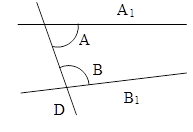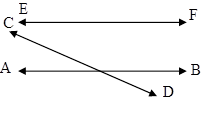Filter By

## All Questions

#### i)Justify whether it is true to say that the following are the nth terms of an AP.2n–3ii)Justify whether it is true to say that the following are the nth terms of an AP.iii)Justify whether it is true to say that the following are the nth terms of an AP.

i)

Solution.

Hence, 2n – 3 is the nth term of an AP because the common difference is the same.

ii)

Solution.

Here the common difference is not the same

Therefore series is not the nth term of an AP.

iii)

Solution.

Here the common difference is not the same

#### i)In which of the following situations, do the lists of numbers involved form an AP? Give reasons for your answers.The fee charged from a student every month by a school for the whole session, when the monthly fee is Rs 400.ii)In which of the following situations, do the lists of numbers involved form an AP? Give reasons for your answers.The fee charged every month by a school from Classes I to XII, when the monthly fee for Class I is Rs 250, and it increases by Rs 50 for the next higher class.iii)In which of the following situations, do the lists of numbers involved form an AP? Give reasons for your answers.The amount of money in the account of Varun at the end of every year when Rs 1000 is deposited at simple interest of 10% per annum.iv)In which of the following situations, do the lists of numbers involved form an AP? Give reasons for your answers.The number of bacteria in a certain food item after each second, when they double in every second.

i)

Solution.

According to the question:

It is clear that the monthly fee charged from students is = 400 Rs.

Hence series is ; 400, 400, 400, 400 ……

the difference of the successive terms is same so It forms an AP with common difference d = 400 – 400 = 0

ii)

Solution.

According to the question:

Fees started Rs. 250 and increased by 50 Rs. from I to XII is

It forms an AP with common difference 50.

iii)

Solution.         We know that: Simple interest = (P × R × T)/100

This is definite form an AP with common difference of SI=100 Rs.

iv)

Solution.

Let the number of bacteria = x

According to the question:

They double in every second.

Since the difference between the successive terms is not same therefore it does not form an AP.

## Crack CUET with india's "Best Teachers"

• HD Video Lectures
• Unlimited Mock Tests
• Faculty Support#### The taxi fare after each km, when the fare is Rs 15 for the first km and Rs. 8 for each additional km, does not form an AP as the total fare (in Rs) after each km is15, 8, 8, 8, ...Is the statement true? Give reasons.

Solution.

Here difference of the successive terms is not same hence it does not form an AP

Therefore given statement is true.

#### Is 0 a term of the AP: 31, 28, 25, ...? Justify your answer.

Solution.

Here he given series is 31, 28, 25, …….

Which is not an integer.

Hence 0 is not a term of this AP.

## Crack NEET with "AI Coach"

• HD Video Lectures
• Unlimited Mock Tests
• Faculty Support#### Two APs have the same common difference. The first term of one AP is 2 and that of the other is 7. The difference between their 10th terms is the same as the difference between their 21stterms, which is the same as the difference between any two corresponding terms. Why?

Solution.

It is given that the first term of an AP is 2 and of other is 7.

it is because when we find the difference between 10th and 21st term it is equal to the a1-b1  which is the difference between first terms.

#### For the AP: –3, –7, –11, ..., can we find directly without actually finding ?Give reasons for your answer.

Solution. Here the given series is

## Crack JEE Main with "AI Coach"

• HD Video Lectures
• Unlimited Mock Tests
• Faculty Support#### Justify whether it is true to say that   forms an A.P.as

Solution.

#### infoexpert21

i)

Solution.

Here the difference of the successive term is same hence it is an AP.

ii)

Solution.

Here the difference successive term is not same. Hence it is not an AP.

iii)

Solution.

Here the difference successive term is not the same. Hence it is not an AP.

iv)

Solution.

Here the difference of the successive term is same. Hence it is an AP.

v)

Solution.

Here the difference between the successive terms is not the same. Hence it is not an AP.

vi)

Solution.

Here the difference of the successive terms is not the same. Hence it is not an AP.

vii)

Solution.

Here the difference of the successive term is the same. Hence it is an AP.

## Crack CUET with india's "Best Teachers"

• HD Video Lectures
• Unlimited Mock Tests
• Faculty Support#### Write whether the following statements are True or False? Justify your answer:Attempts to prove Euclid’s fifth postulate using the other postulates and axioms led to the discovery of several other geometries.

True

Solution:

Euclid’s fifth postulate: if a straight line falling on two straight lines make the interior angles on the same side less than two right angles, the two straight lines, if produced indefinitely, meet on that side on which the angles are less than two right angles.

It means that if the sum of angles ÐA and ÐB in the figure is less than the sum of two right angles then A, B meet on the same side of angle A and B continued indefinitely.All attempts to prove the fifth postulate as a theorem led to great achievement in the creation of several other geometries. These geometries are different from Euclidean geometry and called non-Euclidean geometry.

Hence the given statement is true.

#### Write whether the following statements are True or False? Justify your answer: Two distinct intersecting lines cannot be parallel to the same line.

True

Solution:

Euclid’s fifth postulate: if a straight line falling on two straight lines make the interior angles on the same side less than two right angles, the two straight lines, if produced indefinitely, meet on that side on which the angles are less than two right angles.

Using the above, we can see that two distinct intersecting lines cannot be parallel to the same line.

Here we can take an example: line AB and CD are intersecting. If we take a line EF parallel to AB then EF is not parallel to CD.Hence the given statements is true.